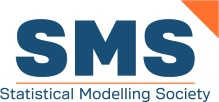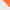Contact# Statistical Modelling Society

www.statmod.orgHomeThe SocietyThe Workshop The IWSM History of the IWSM ArchiveThe JournalNewsletterMembershipMembers OnlyContact Us

## 30th International Workshop on Statistical Modelling, Linz, Austria, 2015, Proceedings

Herwig Friedl and Helga Wagner (Eds.)
Proceedings of the 30th International Workshop on Statistical Modelling. Linz, Austria, 6-10 July 2015

## Contents

### Part I: Invited Papers

Chandler and Ambrosino
Spatiotemporal modelling for environmental applications: challenges and opportunities
Finley et al.
Bayesian functional data models for coupling high-dimensional LiDAR and forest variables over large geographic domains
Frühwirth-Schnatter
Recent advances in sparse Bayesian factor analysis
Greven
A general framework for functional regression
Hinde
All statistical models are wrong, some are usable!

### Part II: Contributed Papers (Volume I)

Ameraoui et al.
Bayesian estimation of the tail index of a heavy tailed distribution with censoring
Bartolucci et al.
Robust maximum likelihood estimation of latent class models
Berger and Tutz
An extended adjacent categories model accounting for response styles
Bottone et al.
Quantile regression: a Bayesian robust approach
Bremhorst et al.
Time dependent variables in cure survival models: An application to the timing of a third pregnancy
Brockhaus et al.
Functional regression models for location, scale and shape applied to stock returns
Camarda and Eilers
Regression models with digit preference
Colombi and Giordano
A mixture model for multidimensional ordinal data
Crespo et al.
Understanding economic growth regressions using latent class analysis
De Mulder et al.
Application of statistical emulation to an agent-based model: assortative mating and the reversal of gender inequality in education in Belgium
Edwards et al.
Signal extraction and power spectral density estimation: A Bayesian semi-parametric approach
Eilers
Separation of signals with tailored penalties
Fallah and Hinde
Modelling homogeneous and heterogenous populations under joint progressive type-II censoring
Amanda Fernández-Fontelo et al.
Generalized INAR models with trend and seasonality for veterinary syndromic surveillance
Frasso et al.
A Bayesian model for the Ebola epidemic in Sierra Leone
Frühwirth-Schnatter and Posekany
Merging parallel MCMC output with yin-yang sampling
Gampe et al.
Hazard modelling for interval-censored data by smoothing within the EM algorithm
Gasparrini et al.
Penalized distributed lag non-linear models
Goerg et al.
How many people visit YouTube? Imputing missing events in panels with excess zeros
Groll et al.
Regularization in Cox frailty models
Grzegorczyk et al.
Network reconstruction with realistic models
Heller et al.
Dispersion modelling for Poisson-inverse Gaussian regression
Hermans et al.
Clusters with random size: maximum likelihood versus weighted estimation
Hofner and Smith
Boosted negative binomial hurdle models for spatiotemporal abundance of sea birds
Hsu et al.
Analysis of accelerated failure time data with dependent censoring
Iannario and Lang
Testing conditional independence in sets of I x J tables
Jaspers et al.
Estimation of an MIC distribution using the vertex exchange method
Kateri and Agresti
Average effect measures for group comparisons in ordinal response models
Klein et al.
Klein and Kneib
Scale-dependent priors for variance parameters in distributional regression
Klingenberg
Multiple comparisons of marginal probabilities following GEE estimation
Kosmidis et al.
Beyond beta regression: modelling percentages and fractions in the presence of boundary observations
Lambert and Denuit
Density estimation from tabulated summary statistics
Larocque and Simonoff
Model-based mixed effects trees for longitudinal and clustered data
Functional forms for transition intensities in a progressive three-state model
Marx
Varying single-index signal regression
Mayr et al.
Boosting the discriminatory power of survival models via the C-index and stability selection
McParland and Gormley
Clustering mixed data via latent variable models
Moral et al.
Assessing goodness-of-fit for accelerated failure rate models: An insect ecology case-study
Oliveira I. et al.
Quantifying the genetic contribution to the variability of count traits
Peyhardi
New qualitative choice models incorporating individual and choice characteristics
Reichl
Estimating marginal likelihoods from the posterior draws through a geometric identity
Rodríguez-Álvarez et al.
Fast estimation of multidimensional adaptive P-spline models
Schauberger and Tutz
BTL-Lasso -- A penalty approach to heterogeneity in paired comparison data
Schneider and Tutz
Variable selection in mixture models for ordinal response with an uncertainty component
Siino et al.
Penalized logistic regression for small or sparse data: interval estimators revisited
da Silva et al.
Doubly robust-based GEE for the analysis of longitudinal ordinal missing data
Spangl et al.
Statistical models for dynamics in extreme value processes
Stauffer et al.
Spatio-temporal censored model of precipitation climatology
Torretta et al.
P-spline quantile regression with a mixed model algorithm
Vana et al.
Modeling creditworthiness using a generalized linear mixed-effects approach
Waldmann and Taylor-Robinson
Joint modelling of quantile regression and survival time of lung function decline in cystic fibrosis patients
Weldon and Titman
Estimation of two-sided choice models: an application to public school choice
Würbach and Zinn
Estimation of a general heaping model via random-walk Metropolis algorithms

### Part III: Contributed Papers (Volume II)

Agogo et al.
Measurement error modelling for accelerometer activity data using Bayesian integrated nested Laplace approximation
Alvares et al.
Bayesian multinomial logit model: an application to agriculture
Anan et al.
Population size estimation in capture-recapture data based upon the Conway-Maxwell-Poisson distribution
Araveeporn
Estimating nonlinear autoregressive models using smoothing spline and penalized spline methods
Bacci et al.
Longitudinal data with informative dropout: an approach based on an AR(1) latent process
Bakka
Log-Gaussian Cox processes with spatially varying second order properties
Banditvilai and Mahakeeta
A receiving process simulation model of the consumer product distribution center
Bigirumurame et al.
Integrated analysis of multi-source data in drug discovery experiments using structural equation models
Bitto and Frühwirth-Schnatter
Achieving shrinkage in the time-varying parameter models framework
Blas
Skew-normal controlled calibration model
Böhnstedt and Gampe
Density estimation from uncertain observations -- Comparing parametric and nonparametric methods
Bollmann et al.
Evaluation of a new k-means approach for exploratory clustering of items
Bruyndonckx et al.
Using generalized estimating equations to study persistence of antimicrobial resistance in respiratory streptococci
Cakmak et al.
Using spatial and land use regression models in investigating the modifying effect of socioeconomic status on the interaction between traffic, air pollution and asthma
Carrasco et al.
Influence diagnostics in errors-in-variables beta regression models
Cavalcante and Blas
Beta calibration model with measurement errors
Corduas
Modelling correlated ordinal data by a copula approach
Dayaratna and Kedem
A probabilistic examination of the efficacy of tort reform via Bayesian semiparametric density ratio modeling
De Bastiani et al.
Local influence on Gaussian spatial linear model with multiple replications
Vera Djordjilović and Chiogna
An improved shrinkage procedure for the estimation of covariance matrices in graphical models
Espendiller and Kateri
Measures of association for 2 x 2 tables with a phi-divergence origin
Fabio et al.
On the derivation of a multivariate gamma model
Fiaccone and Henderson
Dynamic analysis for event history data: Recurrent infant diarrhoea
da Fonseca et al.
Time-varying cointegration via wavelets
Pillay and McColl
Model selection and model averaging using restrictive strategies for imputation in linear models
Yann Guédon
Slope heuristics for multiple change-point models
Guo et al.
Making Bayesian bivariate meta-analysis practice friendly
Hainy and Drovandi
Approximate Bayesian computation for spatial extremes using composite score functions
Hermann and Stehlík
On some issues on statistical analysis for Wilms tumor
Hernández et al.
GAMLSS applied to study bacterial cellulose yield
Higueras et al.
A new inverse regression model and software for radiation biodosimetry
Hossain et al.
Modelling a proportion response variable using generalised additive models for location scale and shape
Iannario and Piccolo
Analysing ordinal categorical data by means of a generalized mixture model
Jalali et al.
Dynamic nomogram in R
Kastner et al.
Dynamic covariance estimation using sparse Bayesian factor stochastic volatility models
Komárková et al.
Model based segmentation of the Czech hospitals according to their longitudinal financial performance in 2007-2011
Lorenzo-Arribas et al.
Malsiner-Walli et al.
Bayesian variable selection in semi-parameteric growth regression
Mameli et al.
Simulated adjustment of the signed scoring rule root statistic
Marcelletti et al.
A flexible bivariate location-scale finite mixture approach to economic growth
Matawie et al.
An approach to determine clusters overlap for K-means clustering
Nonparametric estimation of the survival function for ordered multivariate failure time data: a comparative study
Flexible models in survival analysis: an illustration
Nakamura et al.
The Birnbaum-Saunders generalized-\$t\$ distribution for positive skewed data
Niu et al.
Inference in nonlinear differential equations
Noè et al.
Emulation of ODEs with Gaussian processes
O'Donnell et al.
Within lake clustering of high resolution satellite retrievals - a functional data and clustering approach
O'Hagan
Bayesian model averaging for copula-based estimation of upper tail dependence in loss distributions
Oliveira T. et al.
Generalized linear mixed models applied to overdispersed proportion data in a fungal occurrence study
Oliveira W. et al.
A general class of bivariate regression models for mixed discrete and continuous responses
Ospina-Galindez et al.
Functional regression model of pentadal rainfall of Valle del Cauca (Colombia) in the period (1993-2011), integrating data from meteorological stations and satellite
Pauger and Wagner
A comparison of different priors for sparse Bayesian modelling in regression models with ordinal predictors
Paula and Relvas
Partially linear models with autoregressive symmetric errors
Perrone and Müller
Ds-optimality for discriminating between copula models: a first example
Pinheiro and Ferrari
A comparative review of generalizations of the Gumbel extreme value distribution
Pinheiro et al.
Constrained hypotheses testing via quasi U-statistics and its application to undergraduate performance assessment
Romeo and Meyer
Bayesian approach for modelling bivariate survival data through the PVF copula
Rennies and Kneib
Structural equation models for dealing with spatial confounding
Roth and Lang
Posterior sensitivity of variance priors in Bayesian structured additive regression
Santos and Bolfarine
Analysis of Brazil's presidential election via Bayesian spatial quantile regression
Schäfer and Worton
Modelling wind direction with an application
Schnabel et al.
Quantifying LD decay by quantile regression -- a case study
Schneider et al.
Spatial variation of drivers of agricultural abandonment with spatially boosted models
Shirazi et al.
Self-modeling ordinal model with time invariant covariates -- an application to prostate cancer
Yu et al.
Statistical analysis of spatial distribution in populations of microspecies of Alchemilla L.
Stefanova et al.
Use of genetic relationship matrices in the prediction of breeding values and their accuracy assessment
Sweeney
A modified binomial likelihood model for zero and n-inflated count data
Tough et al.
Conditional weight gain using an external reference
Tovar-Rios et al.
Prior elicitation for estimation in a mixed effect logistic model
Umlauf et al.
A conceptional Lego toolbox for Bayesian distributional regression models
Vandendijck et al.
Geostatistical analysis using K-splines in the geoadditive model
Van Kerckhove et al.
Do contacts over distance follow a power-law distribution? Estimation of the social contact distance kernel
Wilson and Einbeck
A simple and intuitive test for number-inflation or number-deflation